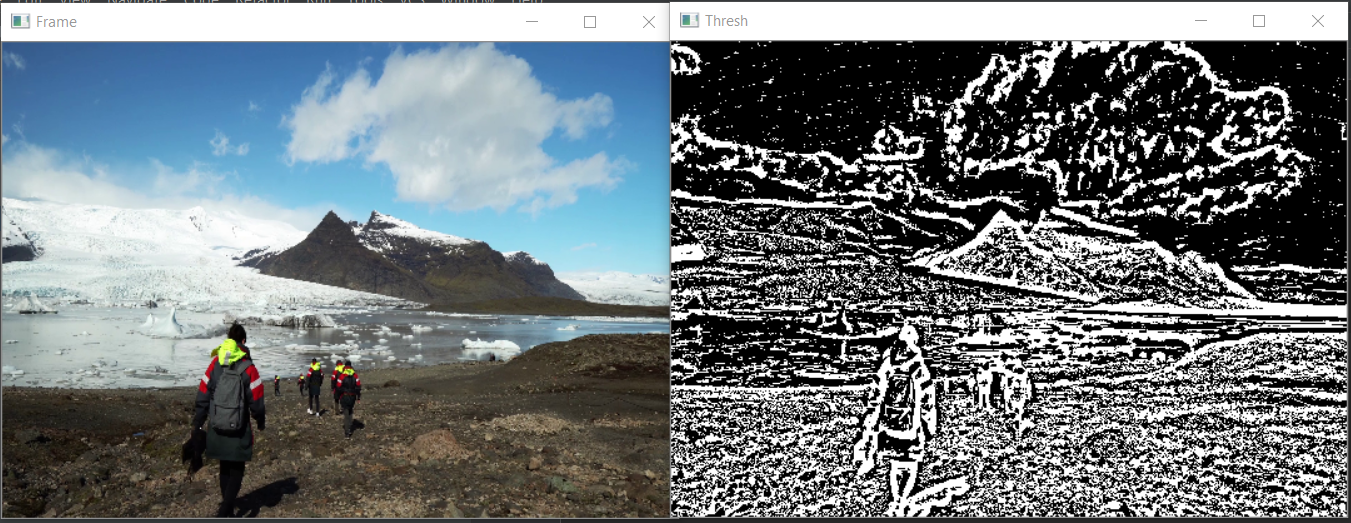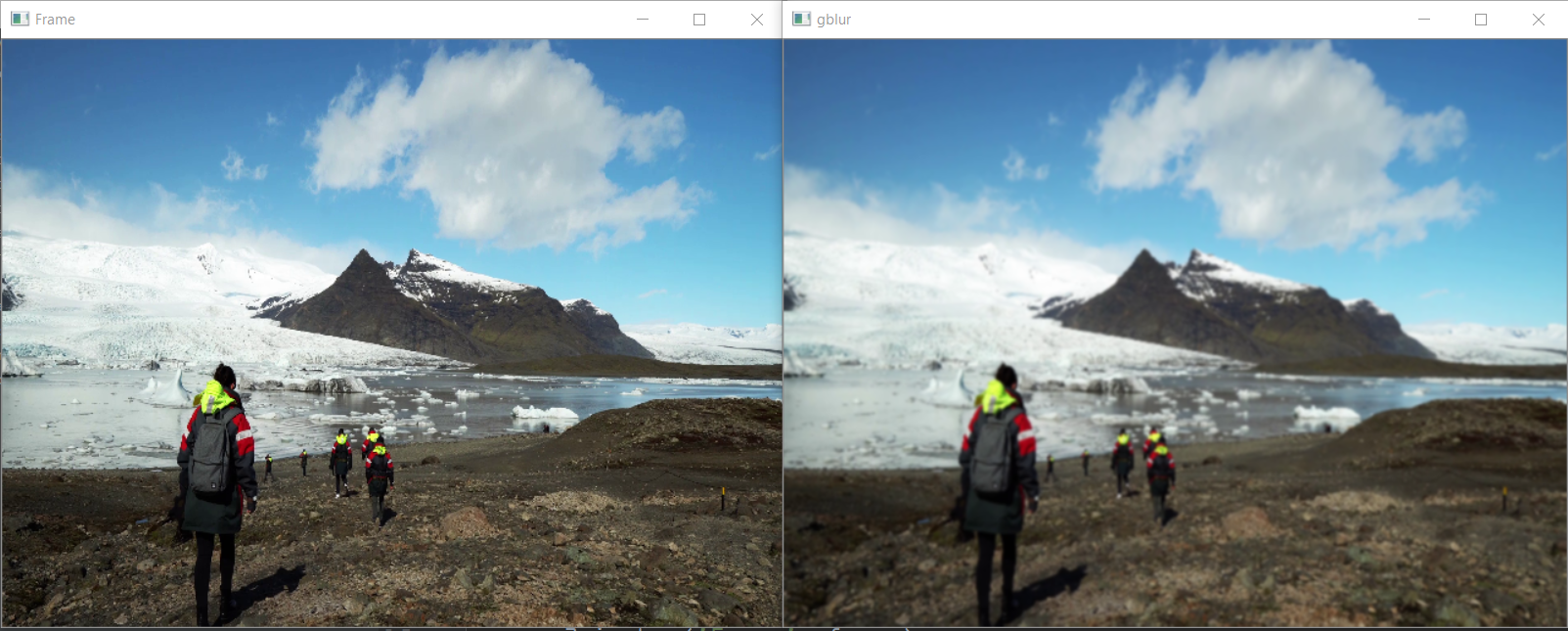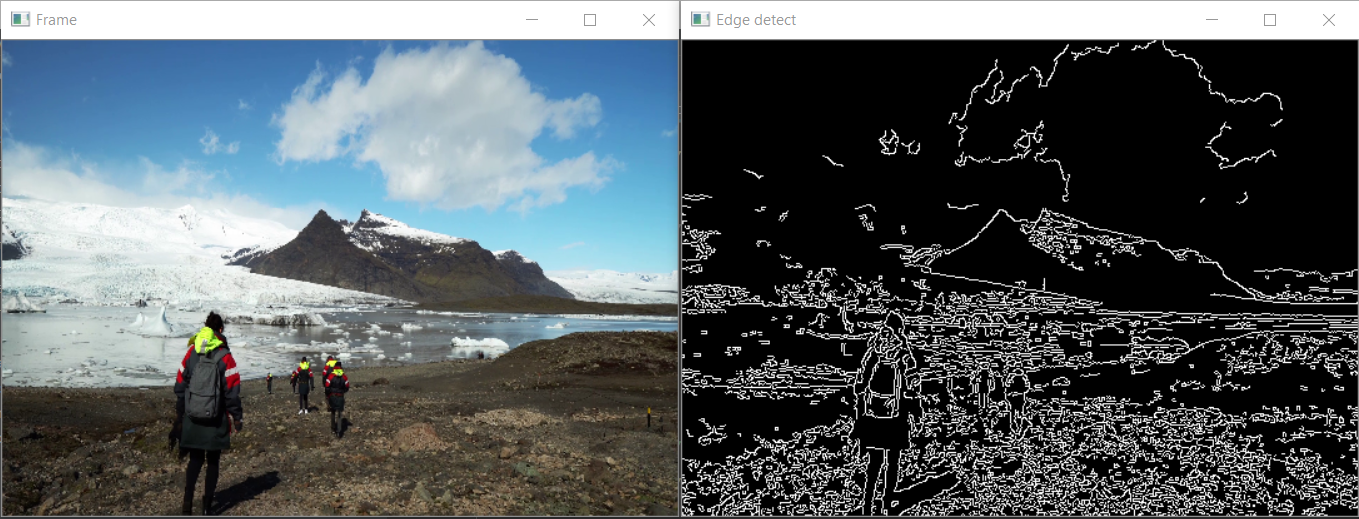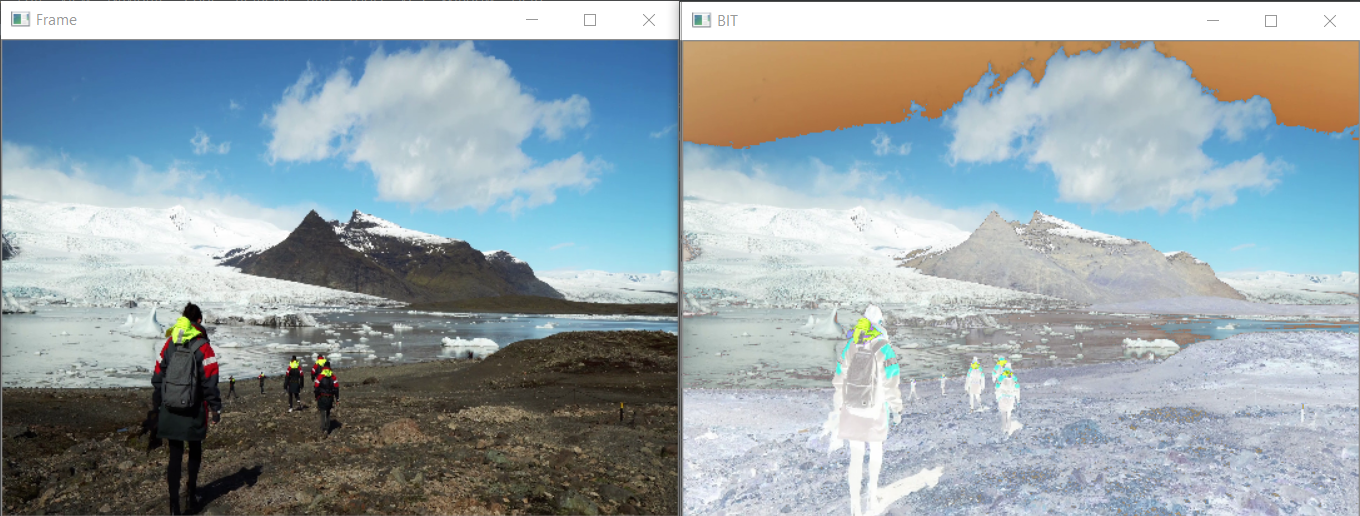# Python – Process images of a video using OpenCV

• Difficulty Level : Expert
• Last Updated : 26 Jul, 2021

Processing a video means, performing operations on the video frame by frame. Frames are nothing but just the particular instance of the video in a single point of time. We may have multiple frames even in a single second. Frames can be treated as similar to an image.
So, whatever operations we can perform on images can be performed on frames as well. Let us see some of the operations with examples.

## Adaptive Threshold –

By using this technique we can apply thresholding on small regions of the frame. So the collective value will be different for the whole frame.

## Python3

 `# importing the necessary libraries` `import` `cv2` `import` `numpy as np`   `# Creating a VideoCapture object to read the video` `cap ``=` `cv2.VideoCapture(``'sample.mp4'``)`     `# Loop until the end of the video` `while` `(cap.isOpened()):`   `    ``# Capture frame-by-frame` `    ``ret, frame ``=` `cap.read()` `    ``frame ``=` `cv2.resize(frame, (``540``, ``380``), fx ``=` `0``, fy ``=` `0``,` `                         ``interpolation ``=` `cv2.INTER_CUBIC)`   `    ``# Display the resulting frame` `    ``cv2.imshow(``'Frame'``, frame)`   `    ``# conversion of BGR to grayscale is necessary to apply this operation` `    ``gray ``=` `cv2.cvtColor(frame, cv2.COLOR_BGR2GRAY)`   `    ``# adaptive thresholding to use different threshold ` `    ``# values on different regions of the frame.` `    ``Thresh ``=` `cv2.adaptiveThreshold(gray, ``255``, cv2.ADAPTIVE_THRESH_MEAN_C,` `                                           ``cv2.THRESH_BINARY_INV, ``11``, ``2``)`   `    ``cv2.imshow(``'Thresh'``, Thresh)` `    ``# define q as the exit button` `    ``if` `cv2.waitKey(``25``) & ``0xFF` `=``=` `ord``(``'q'``):` `        ``break`   `# release the video capture object` `cap.release()` `# Closes all the windows currently opened.` `cv2.destroyAllWindows()`

Output:## Smoothing –

Smoothing a video means removing the sharpness of the video and providing a blurriness to the video. There are various methods for smoothing such as cv2.Gaussianblur(), cv2.medianBlur(), cv2.bilateralFilter(). For our purpose, we are going to use cv2.Gaussianblur().

## Python3

 `# importing the necessary libraries` `import` `cv2` `import` `numpy as np`   `# Creating a VideoCapture object to read the video` `cap ``=` `cv2.VideoCapture(``'sample.mp4'``)`     `# Loop until the end of the video` `while` `(cap.isOpened()):` `    ``# Capture frame-by-frame` `    ``ret, frame ``=` `cap.read()` `    ``frame ``=` `cv2.resize(frame, (``540``, ``380``), fx ``=` `0``, fy ``=` `0``,` `                         ``interpolation ``=` `cv2.INTER_CUBIC)`   `    ``# Display the resulting frame` `    ``cv2.imshow(``'Frame'``, frame)`   `    ``# using cv2.Gaussianblur() method to blur the video`   `    ``# (5, 5) is the kernel size for blurring.` `    ``gaussianblur ``=` `cv2.GaussianBlur(frame, (``5``, ``5``), ``0``) ` `    ``cv2.imshow(``'gblur'``, gaussianblur)`   `    ``# define q as the exit button` `    ``if` `cv2.waitKey(``25``) & ``0xFF` `=``=` `ord``(``'q'``):` `        ``break`   `# release the video capture object` `cap.release()`   `# Closes all the windows currently opened.` `cv2.destroyAllWindows()`

Output:## Edge Detection –

Edge detection is a useful technique to detect he edges of surfaces and objects in the video. Edge detection involves the following steps:

• Noise reduction
• Non-maximum suppression
• Double threshold
• Edge tracking by hysteresis

## Python3

 `# importing the necessary libraries` `import` `cv2` `import` `numpy as np`   `# Creating a VideoCapture object to read the video` `cap ``=` `cv2.VideoCapture(``'sample.mp4'``)`     `# Loop until the end of the video` `while` `(cap.isOpened()):` `    ``# Capture frame-by-frame` `    ``ret, frame ``=` `cap.read()`   `    ``frame ``=` `cv2.resize(frame, (``540``, ``380``), fx ``=` `0``, fy ``=` `0``,` `                         ``interpolation ``=` `cv2.INTER_CUBIC)`   `    ``# Display the resulting frame` `    ``cv2.imshow(``'Frame'``, frame)`   `    ``# using cv2.Canny() for edge detection.` `    ``edge_detect ``=` `cv2.Canny(frame, ``100``, ``200``)` `    ``cv2.imshow(``'Edge detect'``, edge_detect)`   `    ``# define q as the exit button` `    ``if` `cv2.waitKey(``25``) & ``0xFF` `=``=` `ord``(``'q'``):` `        ``break`   `# release the video capture object` `cap.release()` `# Closes all the windows currently opened.` `cv2.destroyAllWindows()`

Output:## Bitwise Operations –

Bitwise operations are useful to mask different frames of a video together. Bitwise operations are just like we have studied in the classroom such as AND, OR, NOT, XOR.

## Python3

 `# importing the necessary libraries` `import` `cv2` `import` `numpy as np`   `# Creating a VideoCapture object to read the video` `cap ``=` `cv2.VideoCapture(``'sample.mp4'``)`     `# Loop until the end of the video` `while` `(cap.isOpened()):` `    ``# Capture frame-by-frame` `    ``ret, frame ``=` `cap.read()` `    ``frame ``=` `cv2.resize(frame, (``540``, ``380``), fx ``=` `0``, fy ``=` `0``, ` `                         ``interpolation ``=` `cv2.INTER_CUBIC)`   `    ``# Display the resulting frame` `    ``cv2.imshow(``'Frame'``, frame)` `    `  `    ``# conversion of BGR to grayscale is necessary to apply this operation` `    ``gray ``=` `cv2.cvtColor(frame, cv2.COLOR_BGR2GRAY)`   `    ``_, mask ``=` `cv2.threshold(gray, ``0``, ``255``, cv2.THRESH_BINARY_INV ``+` `cv2.THRESH_OTSU)`   `    ``# apply NOT operation on image and mask generated by thresholding` `    ``BIT ``=` `cv2.bitwise_not(frame, frame, mask ``=` `mask)` `    ``cv2.imshow(``'BIT'``, BIT)`   `    ``# define q as the exit button` `    ``if` `cv2.waitKey(``25``) & ``0xFF` `=``=` `ord``(``'q'``):` `        ``break`   `# release the video capture object` `cap.release()`   `# Closes all the windows currently opened.` `cv2.destroyAllWindows()`

Output:We can perform any other operations according to our needs. These are just few basic operations that are mostly used.

My Personal Notes arrow_drop_up
Recommended Articles
Page :